# Count Unique Values

This example shows you how to create an array formula that counts unique values.

1. We use the COUNTIF function. For example, to count the number of 5's, use the following function.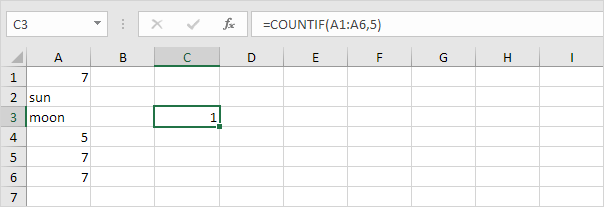2. To count the unique values (don't be overwhelmed), we add the SUM function, 1/, and replace 5 with A1:A6.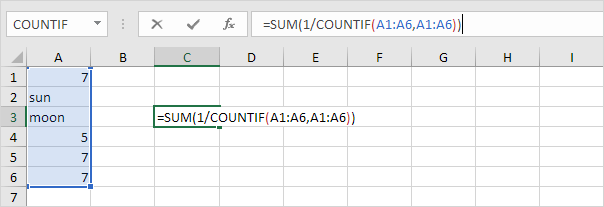3. Finish by pressing CTRL + SHIFT + ENTER.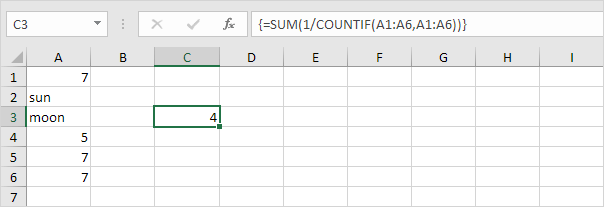Note: The formula bar indicates that this is an array formula by enclosing it in curly braces {}. Do not type these yourself. They will disappear when you edit the formula.

Explanation: The range (array constant) created by the COUNTIF function is stored in Excel's memory, not in a range. The array constant looks as follows:

{3;1;1;1;3;3} - (three 7's, one sun, one moon, one 5, three 7's, three 7's)

This reduces to:
{1/3;1/1;1/1;1/1;1/3;1/3}

This array constant is used as an argument for the SUM function, giving a result of 1/3+1+1+1+1/3+1/3 = 4.

4. The array formula below counts the number of values that occur exactly once.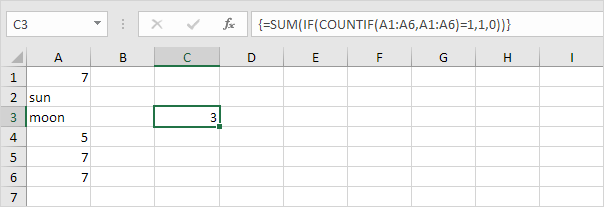Explanation: the IF function converts the array constant {3;1;1;1;3;3} to {0;1;1;1;0;0}. This array constant is used as an argument for the SUM function, giving a result of 3.

5. If you have Excel 365 or Excel 2021, simply use the magic UNIQUE function to extract unique values.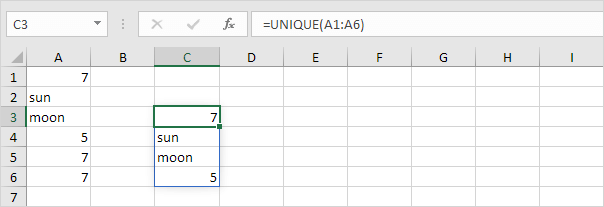Note: this dynamic array function, entered into cell C3, fills multiple cells. Wow! This behavior in Excel 365/2021 is called spilling.

6. Next, add the COUNTA function to count unique values in Excel 365/2021.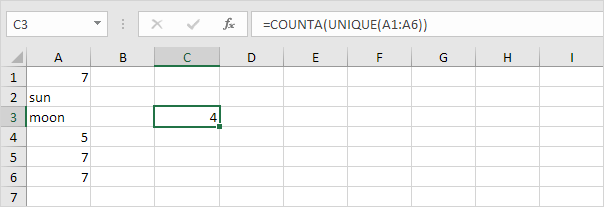7. The formula below counts the number of values that occur exactly once.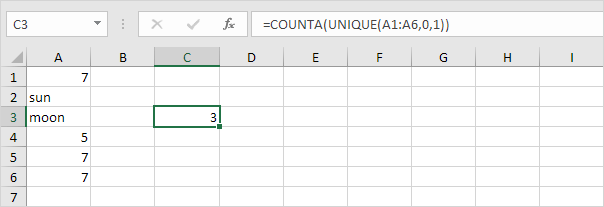Note: the UNIQUE function has 2 optional arguments. The default value of 0 (second argument) tells the UNIQUE function to extract values from a vertical array. The value 1 (third argument) tells the UNIQUE function to extract values that occur exactly once.

Go to Next Chapter: Sort